The average height of a current NBA player is 79 inches with a standard deviation of 3.4 inches. A random sample of 35 current NBA players i

Question

The average height of a current NBA player is 79 inches with a standard deviation of 3.4 inches. A random sample of 35 current NBA players is taken. What is the probability that the mean height of the 35 NBA players will be more than 80 inches?

in progress 0
6 months 2021-07-26T02:17:30+00:00 1 Answers 22 views 0

0.0409 = 4.09% probability that the mean height of the 35 NBA players will be more than 80 inches.

Step-by-step explanation:

To solve this question, we need to understand the normal probability distribution and the central limit theorem.

Normal Probability Distribution

Problems of normal distributions can be solved using the z-score formula.

In a set with mean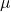and standard deviation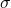, the z-score of a measure X is given by: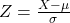The Z-score measures how many standard deviations the measure is from the mean. After finding the Z-score, we look at the z-score table and find the p-value associated with this z-score. This p-value is the probability that the value of the measure is smaller than X, that is, the percentile of X. Subtracting 1 by the p-value, we get the probability that the value of the measure is greater than X.

Central Limit Theorem

The Central Limit Theorem establishes that, for a normally distributed random variable X, with meanand standard deviation, the sampling distribution of the sample means with size n can be approximated to a normal distribution with meanand standard deviation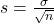.

For a skewed variable, the Central Limit Theorem can also be applied, as long as n is at least 30.

The average height of a current NBA player is 79 inches with a standard deviation of 3.4 inches.

This means that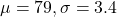A random sample of 35 current NBA players is taken.

This means that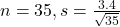What is the probability that the mean height of the 35 NBA players will be more than 80 inches?

This is 1 subtracted by the p-value of Z when X = 80. SoBy the Central Limit Theorem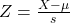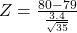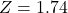has a p-value of 0.9591

1 – 0.9591 = 0.0409

0.0409 = 4.09% probability that the mean height of the 35 NBA players will be more than 80 inches.